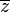# Complex plane facts for kids

Kids Encyclopedia Facts

In algebra and calculus, the complex plane is used to help visualize complex numbers. The vertical line is called the imaginary axis, and the horizontal line is called the real axis. Any complex number can be written as a+bi, where a and b are real numbers, and i is the principal square root of -1. In the complex plane, you go a spaces on the real axis, and b spaces on the imaginary axis. The complex plane can also be expressed in the form of polar coordinates, where the size of the line is equal to the square root of a squared plus b squared, and the direction is an angle.

The complex plane can be used to draw the graphs of some functions, called complex functions, that can be used to study all kinds of things, like cars, airplanes, atoms and electricity.

## Complex conjugates

Every complex number z=a+bi has a complex conjugate. It is written as$\overline{z}$ and is equal to a-bi. The complex number and its conjugate can be represented on the complex plane—as mirror opposites about the real axis.

## Images for kidsComplex plane Facts for Kids. Kiddle Encyclopedia.